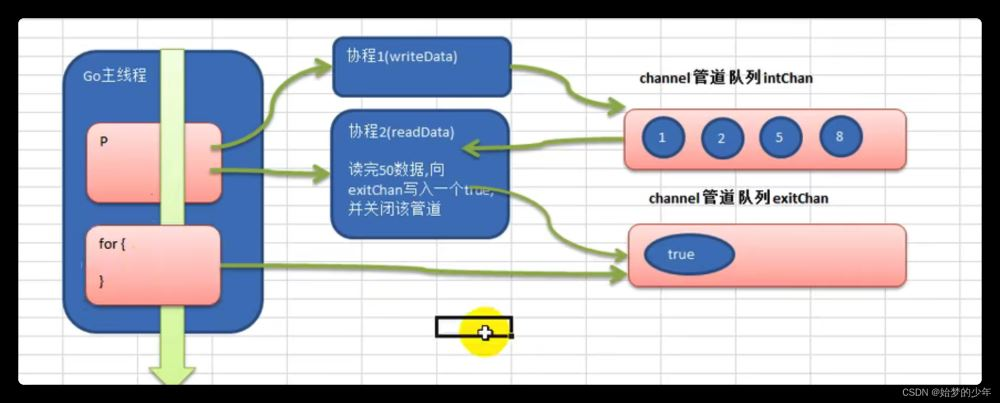﻿ 图文详解Go中的channel_Golang_脚本之家
Golang# 图文详解Go中的channel

Channel是go语言内置的一个非常重要的特性,也是go并发编程的两大基石之一,下面这篇文章主要给大家介绍了关于Go中channel的相关资料,需要的朋友可以参考下

## channel

Go语言中的通道（channel）是一种特殊的类型。

（1）channel本身是一个队列，先进先出

（2）线程安全，不需要加锁

（3）本身是有类型的，string, int 等，如果要存多种类型，则定义成 interface类型

（4）channel是引用类型，必须make之后才能使用，一旦 make，它的容量就确定了，不会动态增加！！它和map，slice不一样

（1）一旦初始化容量，就不会改变了。

（2）当写满时，不可以写，取空时，不可以取。

（3）发送将持续阻塞直到数据被接收

（4）接收将持续阻塞直到发送方发送数据。

（5）每次接收一个元素。

## 1、关于 channel的声明和使用的代码：

```package main
import (
"fmt"
)

func main() {

//演示一下管道的使用
//1. 创建一个可以存放3个int类型的管道
var intChan chan int
intChan = make(chan int, 3)

//2. 看看intChan是什么
fmt.Printf("intChan 的值=%v intChan本身的地址=%p\n", intChan, &intChan)

//3. 向管道写入数据
intChan<- 10
num := 211
intChan<- num
intChan<- 50
// //如果从channel取出数据后，可以继续放入
<-intChan
intChan<- 98//注意点, 当我们给管写入数据时，不能超过其容量

//4. 看看管道的长度和cap(容量)
fmt.Printf("channel len= %v cap=%v \n", len(intChan), cap(intChan)) // 3, 3

//5. 从管道中读取数据

var num2 int
num2 = <-intChan
fmt.Println("num2=", num2)
fmt.Printf("channel len= %v cap=%v \n", len(intChan), cap(intChan))  // 2, 3

num3 := <-intChan
num4 := <-intChan

//num5 := <-intChan

fmt.Println("num3=", num3, "num4=", num4/*, "num5=", num5*/)
}
```
```fmt.Printf("intChan 的值=%v intChan本身的地址=%p\n", intChan, &intChan)
```

## 2、注意空接口类型的 channel

```package main
import (
"fmt"
)

type Cat struct {
Name string
Age int
}

func main() {

//定义一个存放任意数据类型的管道 3个数据
//var allChan chan interface{}
allChan := make(chan interface{}, 3)

allChan<- 10
allChan<- "tom jack"
cat := Cat{"小花猫", 4}
allChan<- cat

//我们希望获得到管道中的第三个元素，则先将前2个推出
<-allChan
<-allChan

newCat := <-allChan //从管道中取出的Cat是什么?

fmt.Printf("newCat=%T , newCat=%v\n", newCat, newCat)
//下面的写法是错误的!编译不通过
//fmt.Printf("newCat.Name=%v", newCat.Name)
//使用类型断言
a := newCat.(Cat)
fmt.Printf("newCat.Name=%v", a.Name)
}
```

a := newCat.(Cat)

## 4、channel的遍历: for … range

1.阻塞接收数据

`data := <-ch`

`data, ok := <-ch`

data：表示接收到的数据。未接收到数据时，data 为通道类型的零值。
ok：表示是否接收到数据。

3.接收任意数据，忽略接收的数据

`<-ch`

```package main

import (
"fmt"
)

func main() {

// 构建一个通道
ch := make(chan int)

// 开启一个并发匿名函数
go func() {

fmt.Println("start goroutine")

// 通过通道通知main的goroutine
ch <- 0

fmt.Println("exit goroutine")

}()

fmt.Println("wait goroutine")

// 等待匿名goroutine
<-ch

fmt.Println("all done")

}
```

4.循环接收

```for data := range ch {
}
```

```package main
import (
"fmt"
)

func main() {

intChan := make(chan int, 3)
intChan<- 100
intChan<- 200
close(intChan) // close
//这时不能够再写入数到channel
//intChan<- 300
fmt.Println("okook~")
//当管道关闭后，读取数据是可以的
n1 := <-intChan
fmt.Println("n1=", n1)

//遍历管道
intChan2 := make(chan int, 100)
for i := 0; i < 100; i++ {
intChan2<- i * 2  //放入100个数据到管道
}

//遍历管道不能使用普通的 for 循环
// for i := 0; i < len(intChan2); i++ {

// }
//在遍历时，如果channel已经关闭，则会正常遍历数据，遍历完后，就会退出遍历
close(intChan2)
for v := range intChan2 { //没有下标
fmt.Println("v=", v)
}
}
``````package main

import (
"fmt"
)

//write Data
func writeData(intChan chan int) {
for i := 1; i <= 50; i++ {
//放入数据
intChan <- i //
fmt.Println("writeData ", i)
//time.Sleep(time.Second)
}
close(intChan) //关闭
}

func readData(intChan chan int, exitChan chan bool) {

for {
v, ok := <-intChan
if !ok {
break
}
// time.Sleep(time.Second)
}
exitChan <- true
close(exitChan)

}

func main() {

//创建两个管道
intChan := make(chan int, 10)
exitChan := make(chan bool, 1)

go writeData(intChan)

//time.Sleep(time.Second * 10)
for {
_, ok := <-exitChan
if !ok {
break
}
}

}

```• intChan ：放8000个数
• primeChan：放素数
• exitChan ：4个协程运行完毕的标志
```package main

import (
"fmt"
"time"
)

//向 intChan放入 1-8000个数
func putNum(intChan chan int) {

for i := 1; i <= 80000; i++ {
intChan <- i
}

//关闭intChan
close(intChan)
}

// 从 intChan取出数据，并判断是否为素数,如果是，就
// 	//放入到primeChan
func primeNum(intChan chan int, primeChan chan int, exitChan chan bool) {

//使用for 循环
// var num int
var flag bool //
for {
//time.Sleep(time.Millisecond * 10)
num, ok := <-intChan //intChan 取不到..

if !ok {
break
}
flag = true //假设是素数
//判断num是不是素数
for i := 2; i < num; i++ {
if num%i == 0 { //说明该num不是素数
flag = false
break
}
}

if flag {
//将这个数就放入到primeChan
primeChan <- num
}
}

//这里我们还不能关闭 primeChan
//向 exitChan 写入true
exitChan <- true

}

func main() {

intChan := make(chan int, 1000)
primeChan := make(chan int, 20000) //放入结果
//标识退出的管道
exitChan := make(chan bool, 4) // 4个

start := time.Now().Unix()

//开启一个协程，向 intChan放入 1-8000个数
go putNum(intChan)
//开启4个协程，从 intChan取出数据，并判断是否为素数,如果是，就
//放入到primeChan
for i := 0; i < 4; i++ {
}

//这里我们主线程，进行处理
//直接
go func() {
for i := 0; i < 4; i++ {
<-exitChan
}

end := time.Now().Unix()
fmt.Println("使用协程耗时=", end-start)

//当我们从exitChan 取出了4个结果，就可以放心的关闭 prprimeChan
close(primeChan)
}()

//遍历我们的 primeChan ,把结果取出
for {
res, ok := <-primeChan
if !ok {
break
}
//将结果输出
fmt.Printf("素数=%d\n", res)
}

fmt.Println("main线程退出")

}
```

main线程退出

```go func() {
for i := 0; i < 4; i++ {
<-exitChan
}

end := time.Now().Unix()
fmt.Println("使用协程耗时=", end-start)

//当我们从exitChan 取出了4个结果，就可以放心的关闭 prprimeChan
close(primeChan)
}()
```

if len(exitChan) == 4